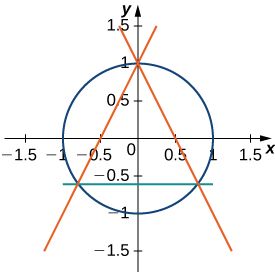## 6.1 Areas between Curves

### Learning Objectives

• Determine the area of a region between two curves by integrating with respect to the independent variable.
• Find the area of a compound region.
• Determine the area of a region between two curves by integrating with respect to the dependent variable.

In Introduction to Integration, we developed the concept of the definite integral to calculate the area below a curve on a given interval. In this section, we expand that idea to calculate the area of more complex regions. We start by finding the area between two curves that are functions of $x,$ beginning with the simple case in which one function value is always greater than the other. We then look at cases when the graphs of the functions cross. Last, we consider how to calculate the area between two curves that are functions of $y.$

# Area of a Region between Two Curves

Let $f(x)$ and $g(x)$ be continuous functions over an interval $\left[a,b\right]$ such that $f(x)\ge g(x)$ on $\left[a,b\right].$ We want to find the area between the graphs of the functions, as shown in the following figure.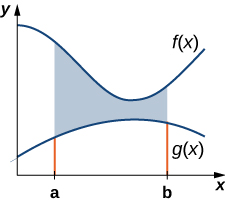Figure 1. The area between the graphs of two functions, $f(x)$ and $g(x),$ on the interval $\left[a,b\right].$

As we did before, we are going to partition the interval on the $x\text{-axis}$ and approximate the area between the graphs of the functions with rectangles. So, for $i=0,1,2\text{,…},n,$ let $P=\left\{{x}_{i}\right\}$ be a regular partition of $\left[a,b\right].$ Then, for $i=1,2\text{,…},n,$ choose a point ${x}_{i}^{*}\in \left[{x}_{i-1},{x}_{i}\right],$ and on each interval $\left[{x}_{i-1},{x}_{i}\right]$ construct a rectangle that extends vertically from $g({x}_{i}^{*})$ to $f({x}_{i}^{*}).$ (Figure)(a) shows the rectangles when ${x}_{i}^{*}$ is selected to be the left endpoint of the interval and $n=10.$ (Figure)(b) shows a representative rectangle in detail.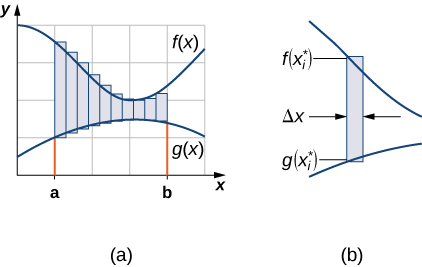Figure 2. (a)We can approximate the area between the graphs of two functions, $f(x)$ and $g(x),$ with rectangles. (b) The area of a typical rectangle goes from one curve to the other.

The height of each individual rectangle is $f({x}_{i}^{*})-g({x}_{i}^{*})$ and the width of each rectangle is $\text{Δ}x.$ Adding the areas of all the rectangles, we see that the area between the curves is approximated by

$A\approx \underset{i=1}{\overset{n}{\text{∑}}}\left[f({x}_{i}^{*})-g({x}_{i}^{*})\right]\text{Δ}x.$

This is a Riemann sum, so we take the limit as $n\to \infty$ and we get

$A=\underset{n\to \infty }{\text{lim}}\underset{i=1}{\overset{n}{\text{∑}}}\left[f({x}_{i}^{*})-g({x}_{i}^{*})\right]\text{Δ}x={\int }_{a}^{b}\left[f(x)-g(x)\right]dx.$

These findings are summarized in the following theorem.

### Finding the Area between Two Curves

Let $f(x)$ and $g(x)$ be continuous functions such that $f(x)\ge g(x)$ over an interval $\left[a,b\right].$ Let $R$ denote the region bounded above by the graph of $f(x),$ below by the graph of $g(x),$ and on the left and right by the lines $x=a$ and $x=b,$ respectively. Then, the area of $R$ is given by

$A={\int }_{a}^{b}\left[f(x)-g(x)\right]dx.$

We apply this theorem in the following example.

### Finding the Area of a Region between Two Curves 1

If R is the region bounded above by the graph of the function $f(x)=x+4$ and below by the graph of the function $g(x)=3-\frac{x}{2}$ over the interval $\left[1,4\right],$ find the area of region $R.$

If $R$ is the region bounded by the graphs of the functions $f(x)=\frac{x}{2}+5$ and $g(x)=x+\frac{1}{2}$ over the interval $\left[1,5\right],$ find the area of region $R.$

#### Hint

Graph the functions to determine which function’s graph forms the upper bound and which forms the lower bound, then follow the process used in (Figure).

In (Figure), we defined the interval of interest as part of the problem statement. Quite often, though, we want to define our interval of interest based on where the graphs of the two functions intersect. This is illustrated in the following example.

### Finding the Area of a Region between Two Curves 2

If $R$ is the region bounded above by the graph of the function $f(x)=9-{(x\text{/}2)}^{2}$ and below by the graph of the function $g(x)=6-x,$ find the area of region $R.$

If R is the region bounded above by the graph of the function $f(x)=x$ and below by the graph of the function $g(x)={x}^{4},$ find the area of region $R.$

#### Hint

Use the process from (Figure).

# Areas of Compound Regions

So far, we have required $f(x)\ge g(x)$ over the entire interval of interest, but what if we want to look at regions bounded by the graphs of functions that cross one another? In that case, we modify the process we just developed by using the absolute value function.

### Finding the Area of a Region between Curves That Cross

Let $f(x)$ and $g(x)$ be continuous functions over an interval $\left[a,b\right].$ Let $R$ denote the region between the graphs of $f(x)$ and $g(x),$ and be bounded on the left and right by the lines $x=a$ and $x=b,$ respectively. Then, the area of $R$ is given by

$A={\int }_{a}^{b}|f(x)-g(x)|dx.$

In practice, applying this theorem requires us to break up the interval $\left[a,b\right]$ and evaluate several integrals, depending on which of the function values is greater over a given part of the interval. We study this process in the following example.

### Finding the Area of a Region Bounded by Functions That Cross

If R is the region between the graphs of the functions $f(x)= \sin x$ and $g(x)= \cos x$ over the interval $\left[0,\pi \right],$ find the area of region $R.$

If R is the region between the graphs of the functions $f(x)= \sin x$ and $g(x)= \cos x$ over the interval $\left[\pi \text{/}2,2\pi \right],$ find the area of region $R.$

#### Hint

The two curves intersect at $x=(5\pi )\text{/}4.$

### Finding the Area of a Complex Region

Consider the region depicted in (Figure). Find the area of $R.$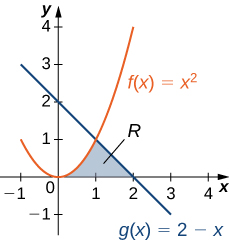Figure 6. Two integrals are required to calculate the area of this region.

Consider the region depicted in the following figure. Find the area of $R.$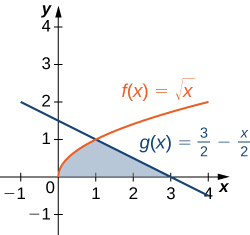#### Hint

The two curves intersect at $x=1.$

# Regions Defined with Respect to $y$

In (Figure), we had to evaluate two separate integrals to calculate the area of the region. However, there is another approach that requires only one integral. What if we treat the curves as functions of $y,$ instead of as functions of $x?$ Review (Figure). Note that the left graph, shown in red, is represented by the function $y=f(x)={x}^{2}.$ We could just as easily solve this for $x$ and represent the curve by the function $x=v(y)=\sqrt{y}.$ (Note that $x=\text{−}\sqrt{y}$ is also a valid representation of the function $y=f(x)={x}^{2}$ as a function of $y.$ However, based on the graph, it is clear we are interested in the positive square root.) Similarly, the right graph is represented by the function $y=g(x)=2-x,$ but could just as easily be represented by the function $x=u(y)=2-y.$ When the graphs are represented as functions of $y,$ we see the region is bounded on the left by the graph of one function and on the right by the graph of the other function. Therefore, if we integrate with respect to $y,$ we need to evaluate one integral only. Let’s develop a formula for this type of integration.

Let $u(y)$ and $v(y)$ be continuous functions over an interval $\left[c,d\right]$ such that $u(y)\ge v(y)$ for all $y\in \left[c,d\right].$ We want to find the area between the graphs of the functions, as shown in the following figure.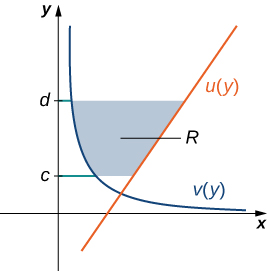Figure 7. We can find the area between the graphs of two functions, $u(y)$ and $v(y).$

This time, we are going to partition the interval on the $y\text{-axis}$ and use horizontal rectangles to approximate the area between the functions. So, for $i=0,1,2\text{,…},n,$ let $Q=\left\{{y}_{i}\right\}$ be a regular partition of $\left[c,d\right].$ Then, for $i=1,2\text{,…},n,$ choose a point ${y}_{i}^{*}\in \left[{y}_{i-1},{y}_{i}\right],$ then over each interval $\left[{y}_{i-1},{y}_{i}\right]$ construct a rectangle that extends horizontally from $v({y}_{i}^{*})$ to $u({y}_{i}^{*}).$ (Figure)(a) shows the rectangles when ${y}_{i}^{*}$ is selected to be the lower endpoint of the interval and $n=10.$ (Figure)(b) shows a representative rectangle in detail.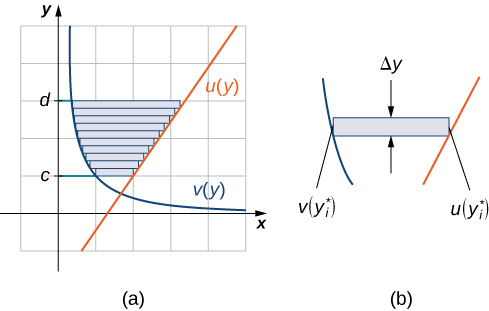Figure 8. (a) Approximating the area between the graphs of two functions, $u(y)$ and $v(y),$ with rectangles. (b) The area of a typical rectangle.

The height of each individual rectangle is $\text{Δ}y$ and the width of each rectangle is $u({y}_{i}^{*})-v({y}_{i}^{*}).$ Therefore, the area between the curves is approximately

$A\approx \underset{i=1}{\overset{n}{\text{∑}}}\left[u({y}_{i}^{*})-v({y}_{i}^{*})\right]\text{Δ}y.$

This is a Riemann sum, so we take the limit as $n\to \infty ,$ obtaining

$A=\underset{n\to \infty }{\text{lim}}\underset{i=1}{\overset{n}{\text{∑}}}\left[u({y}_{i}^{*})-v({y}_{i}^{*})\right]\text{Δ}y={\int }_{c}^{d}\left[u(y)-v(y)\right]dy.$

These findings are summarized in the following theorem.

### Finding the Area between Two Curves, Integrating along the $y$-axis

Let $u(y)$ and $v(y)$ be continuous functions such that $u(y)\ge v(y)$ for all $y\in \left[c,d\right].$ Let $R$ denote the region bounded on the right by the graph of $u(y),$ on the left by the graph of $v(y),$ and above and below by the lines $y=d$ and $y=c,$ respectively. Then, the area of $R$ is given by

$A={\int }_{c}^{d}\left[u(y)-v(y)\right]dy.$

### Integrating with Respect to $y$

Let’s revisit (Figure), only this time let’s integrate with respect to $y.$ Let $R$ be the region depicted in (Figure). Find the area of $R$ by integrating with respect to $y.$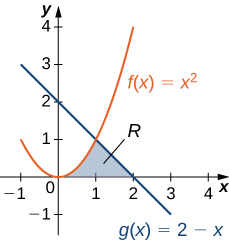Figure 9. The area of region $R$ can be calculated using one integral only when the curves are treated as functions of $y.$

Let’s revisit the checkpoint associated with (Figure), only this time, let’s integrate with respect to $y.$ Let be the region depicted in the following figure. Find the area of $R$ by integrating with respect to $y.$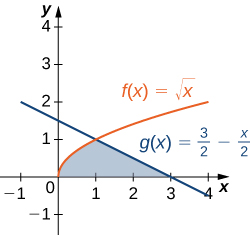#### Hint

Follow the process from the previous example.

### Key Concepts

• Just as definite integrals can be used to find the area under a curve, they can also be used to find the area between two curves.
• To find the area between two curves defined by functions, integrate the difference of the functions.
• If the graphs of the functions cross, or if the region is complex, use the absolute value of the difference of the functions. In this case, it may be necessary to evaluate two or more integrals and add the results to find the area of the region.
• Sometimes it can be easier to integrate with respect to $y$ to find the area. The principles are the same regardless of which variable is used as the variable of integration.

# Key Equations

• Area between two curves, integrating on the $x$-axis
$A={\int }_{a}^{b}\left[f(x)-g(x)\right]dx$
• Area between two curves, integrating on the $y$-axis
$A={\int }_{c}^{d}\left[u(y)-v(y)\right]dy$

For the following exercises, determine the area of the region between the two curves in the given figure by integrating over the $x\text{-axis}\text{.}$

1. $y={x}^{2}-3\text{ and }y=1$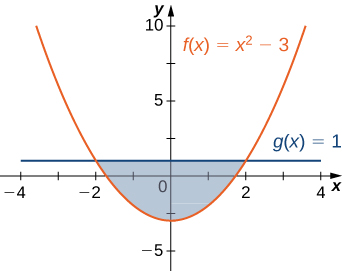2. $y={x}^{2}\text{ and }y=3x+4$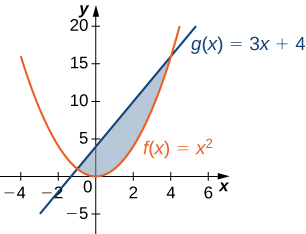For the following exercises, split the region between the two curves into two smaller regions, then determine the area by integrating over the $x\text{-axis}.$ Note that you will have two integrals to solve.

3. $y={x}^{3}$ and $y={x}^{2}+x$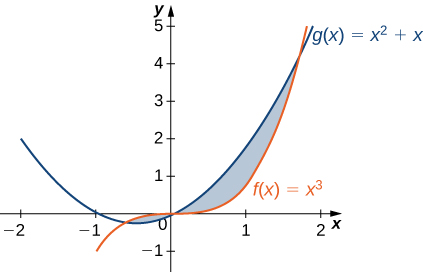4. $y= \cos \theta$ and $y=0.5,$ for $0\le \theta \le \pi$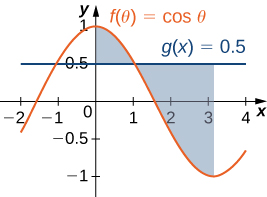For the following exercises, determine the area of the region between the two curves by integrating over the $y\text{-axis}.$

5. $x={y}^{2}\text{ and }x=9$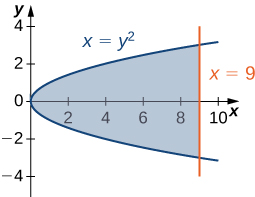6. $y=x\text{ and }x={y}^{2}$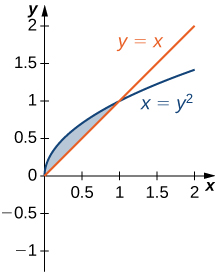For the following exercises, graph the equations and shade the area of the region between the curves. Determine its area by integrating over the $x\text{-axis}.$

7. $y={x}^{2}\text{ and }y=\text{−}{x}^{2}+18x$

8. $y=\frac{1}{x},y=\frac{1}{{x}^{2}},\text{ and }x=3$

9. $y= \cos x$ and $y={ \cos }^{2}x$ on $x=\left[\text{−}\pi ,\pi \right]$

10. $y={e}^{x},y={e}^{2x-1},\text{ and }x=0$

11. $y={e}^{x},y={e}^{\text{−}x},x=-1\text{ and }x=1$

12. $y=e,y={e}^{x},\text{ and }y={e}^{\text{−}x}$

13. $y=|x|\text{ and }y={x}^{2}$

For the following exercises, graph the equations and shade the area of the region between the curves. If necessary, break the region into sub-regions to determine its entire area.

14. $y= \sin (\pi x),y=2x,\text{ and }x>0$

15. $y=12-x,y=\sqrt{x},\text{ and }y=1$

16. $y= \sin x$ and $y= \cos x$ over $x=\left[\text{−}\pi ,\pi \right]$

17. $y={x}^{3}\text{ and }y={x}^{2}-2x$ over $x=\left[-1,1\right]$

18. $y={x}^{2}+9\text{ and }y=10+2x$ over $x=\left[-1,3\right]$

19. $y={x}^{3}+3x$ and $y=4x$

For the following exercises, graph the equations and shade the area of the region between the curves. Determine its area by integrating over the $y\text{-axis}.$

20. $x={y}^{3}\text{ and }x=3y-2$

21. $x=2y\text{ and }x={y}^{3}-y$

22. $x=-3+{y}^{2}\text{ and }x=y-{y}^{2}$

23. ${y}^{2}=x\text{ and }x=y+2$

24. $x=|y|\text{ and }2x=\text{−}{y}^{2}+2$

25. $x= \sin y,x= \cos (2y),y=\pi \text{/}2,\text{ and }y=\text{−}\pi \text{/}2$

For the following exercises, graph the equations and shade the area of the region between the curves. Determine its area by integrating over the $x$-axis or $y$-axis, whichever seems more convenient.

26. $x={y}^{4}\text{ and }x={y}^{5}$

27. $y=x{e}^{x},y={e}^{x},x=0,\text{ and }x=1$

28. $y={x}^{6}\text{ and }y={x}^{4}$

29. $x={y}^{3}+2{y}^{2}+1\text{ and }x=\text{−}{y}^{2}+1$

30. $y=|x|\text{ and }y={x}^{2}-1$

31. $y=4-3x\text{ and }y=\frac{1}{x}$

32. $y= \sin x,x=\text{−}\pi \text{/}6,x=\pi \text{/}6,\text{ and }y={ \cos }^{3}x$

33. $y={x}^{2}-3x+2\text{ and }y={x}^{3}-2{x}^{2}-x+2$

34. $y=2{ \cos }^{3}(3x),y=-1,x=\frac{\pi }{4},\text{ and }x=-\frac{\pi }{4}$

35. $y+{y}^{3}=x\text{ and }2y=x$

36. $y=\sqrt{1-{x}^{2}}\text{ and }y={x}^{2}-1$

37. $y={ \cos }^{-1}x,y={ \sin }^{-1}x,x=-1,\text{ and }x=1$

For the following exercises, find the exact area of the region bounded by the given equations if possible. If you are unable to determine the intersection points analytically, use a calculator to approximate the intersection points with three decimal places and determine the approximate area of the region.

38. [T]$x={e}^{y}\text{ and }y=x-2$

39. [T]$y={x}^{2}\text{ and }y=\sqrt{1-{x}^{2}}$

40. [T]$y=3{x}^{2}+8x+9\text{ and }3y=x+24$

41. [T]$x=\sqrt{4-{y}^{2}}\text{ and }{y}^{2}=1+{x}^{2}$

42. [T]${x}^{2}={y}^{3}\text{ and }x=3y$

43. [T]$y={ \sin }^{3}x+2,y= \tan x,x=-1.5,\text{ and }x=1.5$

44. [T]$y=\sqrt{1-{x}^{2}}\text{ and }{y}^{2}={x}^{2}$

45. [T]$y=\sqrt{1-{x}^{2}}\text{ and }y={x}^{2}+2x+1$

46. [T]$x=4-{y}^{2}\text{ and }x=1+3y+{y}^{2}$

47. [T]$y= \cos x,y={e}^{x},x=\text{−}\pi ,\text{ and }x=0$

48. The largest triangle with a base on the $x\text{-axis}$ that fits inside the upper half of the unit circle ${y}^{2}+{x}^{2}=1$ is given by $y=1+x$ and $y=1-x.$ See the following figure. What is the area inside the semicircle but outside the triangle?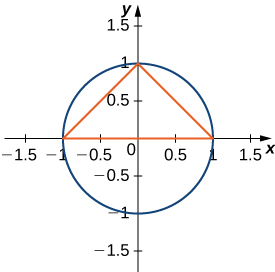49. A factory selling cell phones has a marginal cost function $C(x)=0.01{x}^{2}-3x+229,$ where $x$ represents the number of cell phones, and a marginal revenue function given by $R(x)=429-2x.$ Find the area between the graphs of these curves and $x=0.$ What does this area represent?

50. An amusement park has a marginal cost function $C(x)=1000{e}^{\text{−}x}+5,$ where $x$ represents the number of tickets sold, and a marginal revenue function given by $R(x)=60-0.1x.$ Find the total profit generated when selling 550 tickets. Use a calculator to determine intersection points, if necessary, to two decimal places.

51. The tortoise versus the hare: The speed of the hare is given by the sinusoidal function $H(t)=1- \cos ((\pi t)\text{/}2)$ whereas the speed of the tortoise is $T(t)=(1\text{/}2){ \tan }^{-1}(t\text{/}4),$ where $t$ is time measured in hours and the speed is measured in miles per hour. Find the area between the curves from time $t=0$ to the first time after one hour when the tortoise and hare are traveling at the same speed. What does it represent? Use a calculator to determine the intersection points, if necessary, accurate to three decimal places.

52. The tortoise versus the hare: The speed of the hare is given by the sinusoidal function $H(t)=(1\text{/}2)-(1\text{/}2) \cos (2\pi t)$ whereas the speed of the tortoise is $T(t)=\sqrt{t},$ where $t$ is time measured in hours and speed is measured in kilometers per hour. If the race is over in 1 hour, who won the race and by how much? Use a calculator to determine the intersection points, if necessary, accurate to three decimal places.

For the following exercises, find the area between the curves by integrating with respect to $x$ and then with respect to $y.$ Is one method easier than the other? Do you obtain the same answer?

53. $y={x}^{2}+2x+1\text{ and }y=\text{−}{x}^{2}-3x+4$

54. $y={x}^{4}\text{ and }x={y}^{5}$

55. $x={y}^{2}-2\text{ and }x=2y$

For the following exercises, solve using calculus, then check your answer with geometry.

56. Determine the equations for the sides of the square that touches the unit circle on all four sides, as seen in the following figure. Find the area between the perimeter of this square and the unit circle. Is there another way to solve this without using calculus?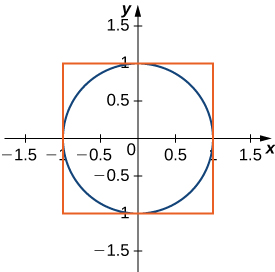57. Find the area between the perimeter of the unit circle and the triangle created from $y=2x+1,y=1-2x$ and $y=-\frac{3}{5},$ as seen in the following figure. Is there a way to solve this without using calculus?